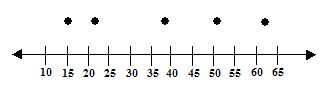Email us to get an instant 20% discount on highly effective K-12 Math & English kwizNET Programs!

#### Online Quiz (WorksheetABCD)

Questions Per Quiz = 2 4 6 8 10

### Grade 7 - Mathematics10.1 Making a Box-and-Whisker Plot

Example:
Use the given data to make a box-and-whisker plot.
23 16 51 23 56 22 63 51 22 15 19 42 44 50 38 31 47

Solution:

1. Order the data and find the smallest value, first quartile, median, third quartile, and largest value
 15, 16, 19, 22, 22, 23, 23, 31, 38, 42, 44, 47, 50, 51, 51, 56, 63

• smallest value: 15
• first quartile: (22+22)/2 = 22
• median: 38
• third quartile: (50+51)/2 = 50.5
• largest value: 63
2. Draw a number line and plot a point about each value from step 13. Draw the box and whiskersDirections: Draw a box-and-whisker plot for the following:
• Math test scores for the class: 80, 75, 90, 95, 65, 65, 80, 85, 70, 100
• Minimum value: 65, first quartile: 70, second quartile (median): 80, third quartile: 90, maximum:100
• The following set of numbers are the amount of pencils fifteen different students own. (The data is already arranged from least to greatest.) 18 27 34 52 54 59 61 68 78 82 85 87 91 93 100
• 4.3, 5.1, 3.9, 4.5, 4.4, 4.9, 5.0, 4.7, 4.1, 4.6, 4.4, 4.3, 4.8, 4.4, 4.2, 4.5, 4.4